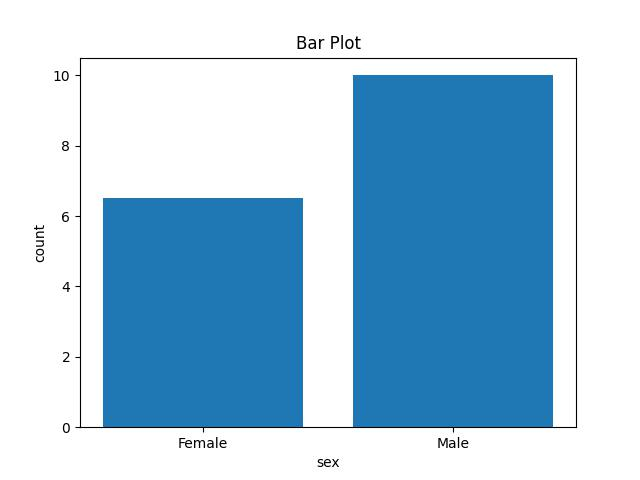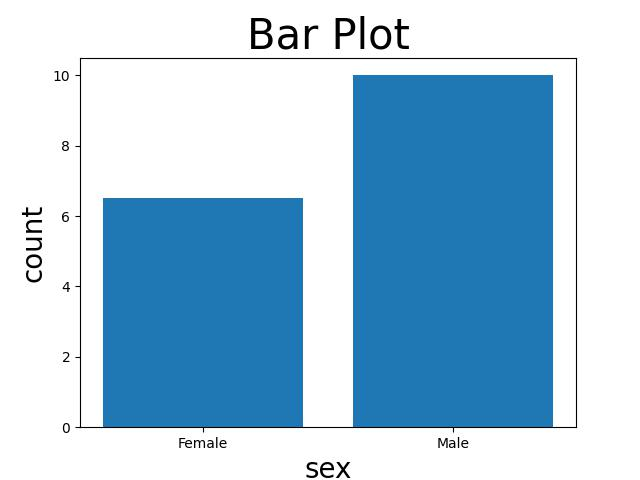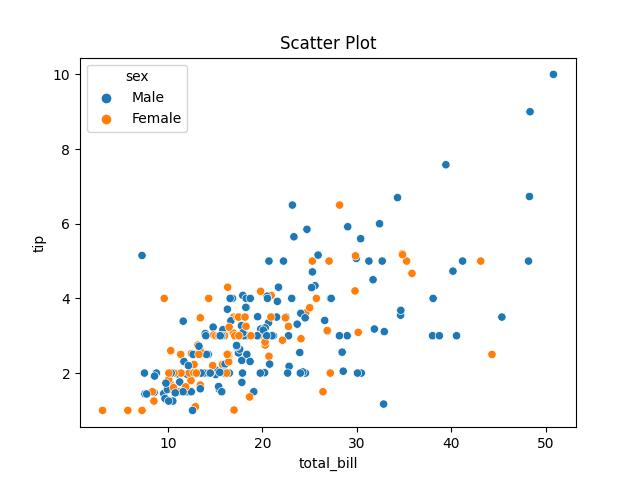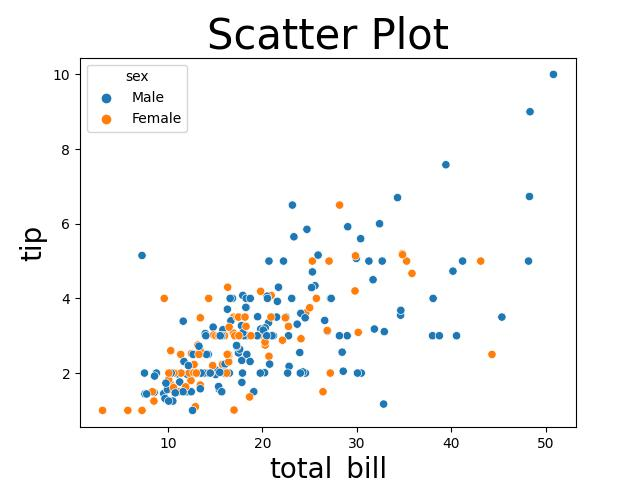# Change Font Size of Plot in Python Matplotlib & seaborn (2 Examples)

Hi! This tutorial will show you how to adjust the font size of a plot in Matplotlib and seaborn in the Python programming language.

Here is an overview:

Let’s get into the Python code!

## Install & Import Matplotlib & seaborn Libraries

If you do not have Matplotlib and seaborn already installed and imported in your Python programming environment, then in your preferred Python IDE, run the lines of code below to install and import Matplotlib and seaborn:

```# install Matplotlib & seaborn
pip install matplotlib seaborn

# import Matplotlib & seaborn
import matplotlib.pyplot as plt
import seaborn as sns```

Now that we have installed and imported both libraries, we can access their plot-building functions, including the ones we will use in this tutorial.

But before we build plots, we need to load the dataset that we will visualize.

We will make use of the tips dataset that comes pre-loaded in seaborn. Therefore, to load the dataset, run the line of code below:

`df = sns.load_dataset("tips")`

You can preview the first 10 rows as follows:

```print(df.head(10))

#    total_bill   tip     sex smoker  day    time  size
# 0       16.99  1.01  Female     No  Sun  Dinner     2
# 1       10.34  1.66    Male     No  Sun  Dinner     3
# 2       21.01  3.50    Male     No  Sun  Dinner     3
# 3       23.68  3.31    Male     No  Sun  Dinner     2
# 4       24.59  3.61  Female     No  Sun  Dinner     4
# 5       25.29  4.71    Male     No  Sun  Dinner     4
# 6        8.77  2.00    Male     No  Sun  Dinner     2
# 7       26.88  3.12    Male     No  Sun  Dinner     4
# 8       15.04  1.96    Male     No  Sun  Dinner     2
# 9       14.78  3.23    Male     No  Sun  Dinner     2```

So, with the dataset loaded, let us now build plots in both Matplotlib and seaborn to demonstrate how to modify the font size of the plots.

## Example 1: Modify Font Size of Plot in Matplotlib

In this example, we will build a basic bar plot with a plot title and axes labels:

```plt.bar(df["sex"],df["tip"])

plt.title("Bar Plot")

plt.xlabel("sex")

plt.ylabel("tips")

plt.show()```In the above example, we built a simple bar plot counting the number of tips given by gender with the plt.bar() function wherein we parsed the “sex” column of the tips DataFrame to the x-axis and the “tips” column to the y-axis. We also set the plot title, and the x and y axes labels using plt.title(), plt.xlabel(), and plt.ylabel() respectively.

Next, we are going to demonstrate how to adjust the font size of the plot:

```plt.bar(df["sex"],df["tip"])

plt.title("Bar Plot",fontsize = 30)

plt.xlabel("sex",fontsize = 20)

plt.ylabel("tips",fontsize = 20)

plt.show()```As you can see in the plot above, the font size of the plot title and labels have been modified. In the functions `plt.title()`, `plt.xlabel()`, and `plt.ylabel()`, we defined the `fontsize =` argument by specifying what size we want the fonts of the plot title and axes labels to be.

## Example 2: Modify Font Size of Plot in seaborn

In this example, we will build a simple scatter plot with a plot title and default axes labels. Run the lines of code below to build the plot:

```sns.scatterplot(df,x = "total_bill",y = "tip",hue = "sex")

plt.title("Scatter Plot")

plt.show()```In the above example, we used the sns.scatterplot() function to build the scatter plot by parsing the DataFrame object to the function and defining the x and y axes of the plot as “total_bill” and “tip” respectively. We also colored the data points by “sex”.

Now, we will demonstrate how to adjust the font size of the plot labels. Therefore, run the lines of code below to do so:

```sns.scatterplot(df,x = "total_bill",y = "tip",hue = "sex")

plt.title("Scatter Plot",fontsize = 30)

plt.xlabel("total bill",fontsize = 20)

plt.ylabel("tip", fontsize = 20)

plt.show()```Since seaborn is a library built on top of Matplotlib, we can use the same functions to alter the font size of the plot, just as we did in the Matplotlib example.

In the `plt.title()` function, we set the font size to 30, while in the `plt.xlabel()` and `plt.ylabel()` functions, we set the font size to 20 just as we did in the Matplotlib example.

## Video, Further Resources & Summary

Do you need more explanations on how to change the font size of a plot in Python Matplotlib and seaborn? Then you should have a look at the following YouTube video of the Statistics Globe YouTube channel.

In the video, we explain in some more detail how to change the font size of a plot in Python Matplotlib and seaborn.

So, with these examples, we have demonstrated in this tutorial how to change the font size of a plot in Python Matplotlib and seaborn. I do hope you found this tutorial helpful!

This post has shown how to change the font size of a plot in Python Matplotlib and seaborn. In case you have further questions, you may leave a comment below.

In the present tutorial, we have adjusted the font size of a bar plot and a scatterplot. However, you may use the same syntax to change the font size of other types of plots such as boxplots, histograms, heatmaps, density plots, and so on.

This page was created in collaboration with Ifeanyi Idiaye. You might check out Ifeanyi’s personal author page to read more about his academic background and the other articles he has written for the Statistics Globe website.

Subscribe to the Statistics Globe Newsletter